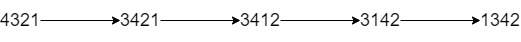# 最多 K 次交换相邻数位后得到的最小整数

## 1505. 最多 K 次交换相邻数位后得到的最小整数 (Hard)```输入：num = "4321", k = 4

```

```输入：num = "100", k = 1

```

```输入：num = "36789", k = 1000

```

```输入：num = "22", k = 22

```

```输入：num = "9438957234785635408", k = 23

```

• `1 <= num.length <= 30000`
• `num` 只包含 数字 且不含有 前导 0
• `1 <= k <= 10^9`

[贪心算法]